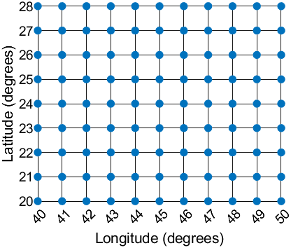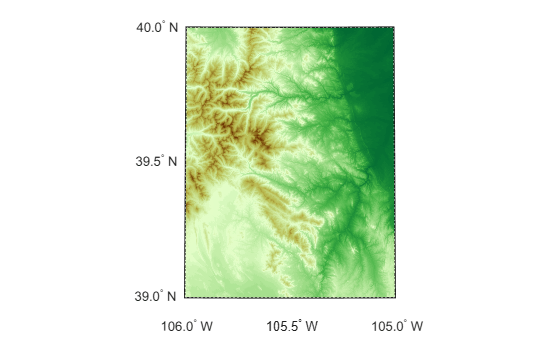# GeographicPostingsReference

Reference raster postings to geographic coordinates

## Description

A geographic postings raster reference object contains information that describes the relationship between a geographic coordinate system and an intrinsic coordinate system that is anchored to the columns and rows of a 2-D spatially referenced grid of point samples (or postings).

In order to reference a raster of postings to geographic coordinates by using a `GeographicPostingsReference` object, the raster must be sampled regularly in latitude and longitude, and its columns and rows must be aligned with meridians and parallels, respectively. The sample spacing from row to row need not equal the sample spacing from column to column.

This image illustrates a 9-by-11 geographic raster of postings. The postings are spaced 1 degree apart in latitude and longitude, cover longitude values in the range [40,50] in degrees, and cover latitude values in the range [20,28] in degrees. The boundary of the raster of postings is a geographic quadrangle with edges that pass through the outermost postings.To reference a raster of cells to geographic coordinates, use a `GeographicCellsReference` object instead.

## Creation

You can use these functions to create `GeographicPostingsReference` objects:

## Properties

expand all

Latitude limits of the geographic quadrangle bounding the georeferenced raster, specified as a two-element vector of the form `[southern_limit northern_limit]`.

Example: `[-20 70]`

Data Types: `double`

Longitude limits of the geographic quadrangle bounding the georeferenced raster, specified as a two-element vector of the form `[western_limit eastern_limit]`.

Example: `[-100 180]`

Data Types: `double`

Number of rows and columns of the raster or image associated with the referencing object, specified as a two-element vector, [m n], where m represents the number of rows and n the number of columns. For convenience, you can assign a size vector having more than two elements. This enables assignments like `R.RasterSize = size(RGB)`, where `RGB` is m-by-n-by-3. In cases like this, the object stores only the first two elements of the size vector and ignores the higher (nonspatial) dimensions.

Example: `[200 300]`

Data Types: `double`

Geometric nature of the raster, specified as `'postings'`.

The value `'postings'` indicates that the raster comprises a grid of sample points, where rows or columns of samples run along the edge of the grid. For an m-by-n raster, points with an intrinsic x-coordinate of 1 or n and/or an intrinsic y-coordinate of 1 or m fall right on an edge (or corner) of the raster.

Data Types: `char`

Unit of measurement used for angle-valued properties, specified as `'degree'`.

Data Types: `char`

Edge from which column indexing starts, specified as `'south'` or `'north'`.

Example: `'south'`

Data Types: `char`

Edge from which row indexing starts, specified as `'west'` or `'east'`.

Example: `'east'`

Data Types: `char`

North-south distance in latitude between adjacent samples (postings) in the raster, specified as a positive numeric scalar. The value is always positive, and is the constant throughout the raster.

Example: `2.5`

Data Types: `double`

East-west distance in longitude between adjacent samples (postings) in the raster, specified as a positive numeric scalar. The value is always positive, and is the constant throughout the raster.

Example: `2.5`

Data Types: `double`

Latitude extent ("height") of the quadrangle covered by the raster, specified as a positive numeric scalar.

Data Types: `double`

Longitude extent ("width") of the quadrangle covered by the raster, specified as a positive numeric scalar.

Data Types: `double`

Raster limits in intrinsic x coordinates, specified as a two-element row vector of positive integers, ```[xMin xMax]```. For an m-by-n raster, `XIntrinsicLimits` equals [1 m], because the `RasterInterpretation` is `'postings'`.

Data Types: `double`

Raster limits in intrinsic y coordinates, specified as a two-element row vector of positive integers, ```[yMin yMax]```. For an m-by-n raster, `YIntrinsicLimits` equals [1 m], because the `RasterInterpretation` is `'postings'`.

Data Types: `double`

Type of coordinate system to which the image or raster is referenced, specified as `'geographic'`.

Data Types: `char`

Geographic coordinate reference system (CRS), specified as a `geocrs` object. A geographic CRS consists of a datum (including its ellipsoid), prime meridian, and angular unit of measurement.

## Object Functions

 `contains` Determine if geographic or map raster contains points `geographicGrid` Geographic coordinates of raster elements `geographicToDiscrete` Transform geographic to discrete coordinates `geographicToIntrinsic` Transform geographic to intrinsic coordinates `intrinsicToGeographic` Transform intrinsic to geographic coordinates `intrinsicXToLongitude` Convert from intrinsic x to longitude coordinates `intrinsicYToLatitude` Convert from intrinsic y to latitude coordinates `latitudeToIntrinsicY` Convert from latitude to intrinsic y coordinates `longitudeToIntrinsicX` Convert from longitude to intrinsic x coordinates `sizesMatch` Determine if geographic or map raster object and image or raster are size-compatible `worldFileMatrix` Return world file parameters for transformation

## Examples

collapse all

Import elevation data as an array and a `GeographicPostingsReference` object by using the `readgeoraster` function. Prepare the data for plotting by specifying the output type as `'double'`.

`[Z,R] = readgeoraster('n39_w106_3arc_v2.dt1','OutputType','double');`

View the properties of the `GeographicPostingsReference` object.

`R`
```R = GeographicPostingsReference with properties: LatitudeLimits: [39 40] LongitudeLimits: [-106 -105] RasterSize: [1201 1201] RasterInterpretation: 'postings' ColumnsStartFrom: 'north' RowsStartFrom: 'west' SampleSpacingInLatitude: 1/1200 SampleSpacingInLongitude: 1/1200 RasterExtentInLatitude: 1 RasterExtentInLongitude: 1 XIntrinsicLimits: [1 1201] YIntrinsicLimits: [1 1201] CoordinateSystemType: 'geographic' GeographicCRS: [1x1 geocrs] AngleUnit: 'degree' ```

Create map axes with limits that match the limits of the reference object. Display the elevation data as a surface. Apply a colormap that is appropriate for elevation data.

```usamap(R.LatitudeLimits,R.LongitudeLimits) geoshow(Z,R,'DisplayType','surface') demcmap(Z)```expand all

Introduced in R2013b

## Support평가판 신청